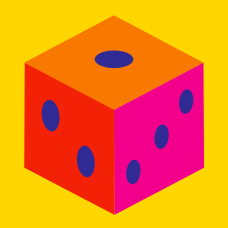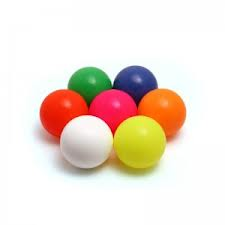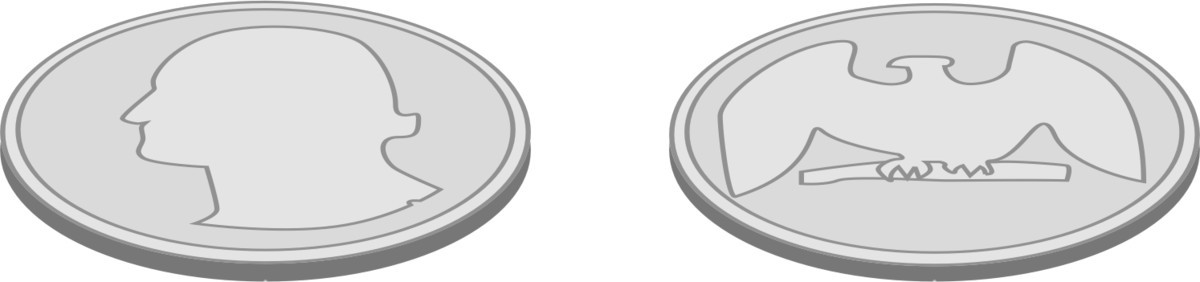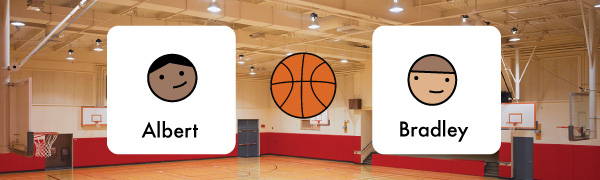Probability

# Discrete Probability: Level 1 ChallengesIn a box, there are 8 red, 7 blue and 6 green balls. One ball is picked up randomly. What is the probability that it is neither red nor green?If two fair coins are tossed simultaneously, what is the probability that exactly one head appears?

Approximately what proportion of the human population was born on February 29th?

Three integers are chosen at random without replacement from the first 20 positive integers.

What is the probability that their product is even?Albert and Bradley can't decide who will get the ball first in a game of basketball. Cathy decides to step in and tell them to pick an integer between $1$ and $100,$ inclusive. Whoever is closer to the number Cathy randomly picked will get the ball first.

Albert randomly guesses the number $37.$ Bradley then picks a number that will maximize his chances of winning. What number did Bradley pick?

×